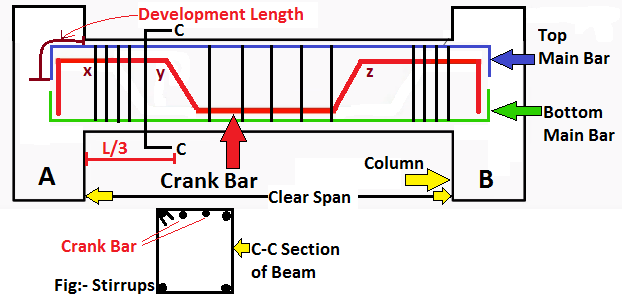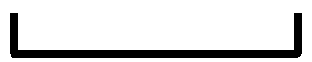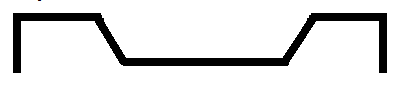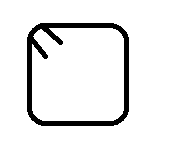# Bar bending schedule of Crank Beam Calculator

## Inter Details of Beammm

mm

mm

feet

x Dia.

mm

……………………………………………….

mm

Nos.

mm

Nos.

mm

Nos.

……………………………………………….

mm

mm

mm

……………………………………………….

Nos.

### Bar Bending Schedule Results

Shape Dia.(mm) No. Cutting Length per Piece(feet) Total Length(feet) Total Weight Required (Kg)
Top Main BarBottom Main BarCrank BarStirrupsThis Calculator calculates the cutting length, the total length and the total weight of a crank bar beam having a single span. It helps you to prepare the bar bending schedule of the column.

## General Formula to calculate the cutting length of any steel bars:

Cutting Length of bars = Perimeter of Shape + Total
hook length – Total Bend Length

## Standards value of Bends & Hooks usedin construction

➢ 1 Hook length = 9d or 75mm
➢ 45° Bend length = 1d
➢ 90° Bend length = 2d
➢ 135° Bend length = 3d
Remember, d = Diameter of Bar

## Steps involved in finding the cutting length of stirrups:-

1. First of write the dimension of the structure.
2. Note down the diameter of steel bars for which
cutting length is to be calculated.
3. Now, deduct the concrete cover or clear cover to get
actual length of perimeter bars.
4. Calculate the length of the hook of the bars.
5. Calculate the bend length of steel bars.
6. Add step 3 and 4 and subtract step 5 from it.
7. It will gives you total cutting length of steel bars.

#### Formula to calculate the weight of steel rod whose length is in foot

Weight = (d2*L)/533, where d is the diameter in millimetres and L is the length of the rod in the foot.